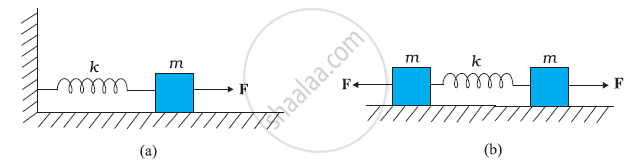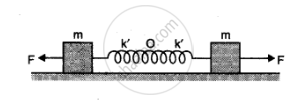CBSE (Science) Class 11CBSE
Share

# Figure (A) Shows a Spring of Force Constant K Clamped Rigidly at One End and a Mass M Attached to Its Free End. a Force F Applied at the Free End Stretches the Spring. Figure (B) Shows the Same Spring with Both Ends Free and Attached to a Mass Mat Either End. Each End of the Spring in Fig. (B) is Stretched by the Same Force - CBSE (Science) Class 11 - Physics

ConceptSimple Harmonic Motion and Uniform Circular Motion

#### Question

Figure (a) shows a spring of force constant clamped rigidly at one end and a mass attached to its free end. A force F applied at the free end stretches the spring. Figure (b) shows the same spring with both ends free and attached to a mass mat either end. Each end of the spring in Fig. (b) is stretched by the same force F.(a) What is the maximum extension of the spring in the two cases?

(b) If the mass in Fig. (a) and the two masses in Fig. (b) are released, what is the period of oscillation in each case?

#### Solution 1

a) For the one block system:

When a force F, is applied to the free end of the spring, an extension l, is produced. For the maximum extension, it can be written as:

F = kl

Where, k is the spring constant

Hence, the maximum extension produced in the spring, l = F/k

For the two block system:

The displacement (x) produced in this case is:

x = l/2

Net force, F = +2 kx = 2k l/2

:. l = F/k

b) For the one block system:

For mass (m) of the block, force is written as:

F = ma = m (d^2x)/dt^2

Where, x is the displacement of the block in time t

:. m (d^2x)/(dt^2) = -kx

It is negative because the direction of elastic force is opposite to the direction of displacement.

(d^2x)/(dt^2) = - (k/m) x = - omega^2x

Where, omega^2 = k/m

Where

ω is angular frequency of the oscillation

:. Time period of the oscillation, T = (2pi)/omega

= (2pi)/sqrt(k/m) = 2pi sqrt(m/k)

For the two block system:

F = m (d^2x)/(dt^2)

m (d^2x)/(dt^2) = - 2kx

where

Angular frequency, omga = sqrt((2k)/m)

:. Time period, T = (2pi)/omega =( 2pi)sqrt(m/(2k))

#### Solution 2

a) Let y be the maximum extension produced in the spring in figure a

Then F = ky (in magnitude)

:. y = F/k

if figure (b) the force on one mass acts as the force of reaction due to the force on the other mass. Therefore, each mass behaves as if it is fixed with respect to the other.

Therefore F= ky => y = F/k

b) In figure (a) F= -ky

=> ma = -ky => a  = k/m y

:.omega^2 = k/m i.e omega = sqrt(k/m)

Therefore, period T = (2pi)/omega = 2pi sqrt(m/k)

In figure (b) we may consider that the centre of the system is O and there are two springs each of length l/2 attached to the two masses, each m, so that k' is the spring factor of each of the springs

Then, K'  =  2k

:. T = 2pi sqrt(m/k')

= 2pi sqrt(m/2k)Is there an error in this question or solution?

#### APPEARS IN

Solution Figure (A) Shows a Spring of Force Constant K Clamped Rigidly at One End and a Mass M Attached to Its Free End. a Force F Applied at the Free End Stretches the Spring. Figure (B) Shows the Same Spring with Both Ends Free and Attached to a Mass Mat Either End. Each End of the Spring in Fig. (B) is Stretched by the Same Force Concept: Simple Harmonic Motion and Uniform Circular Motion.
S